• 一些编辑器最后生成的公式都是图片的样式，我们复制粘贴不出来具体的样式。我们需要用这个插件来转化图片公式
• 写了一个图片的公式。然后直接使用插件把他转化为文本的公式的
• 将latex表示的数学公式转换为图片 基于python实现 图片格式可以任意指定
• latex2word：将Latex（MathJax）转换为MathML（数学标记），以导入到MS Word公式中（作为纯文本）HTML
• 下面介绍的是在vue里面使用的方法： 这是个图片的公式，有img标签引入。 我们想要成下面的效果： 在vue项目中引入MathJax.js 首先在index.html页面 <script type="text/jav...

下面介绍的是在vue里面使用的方法：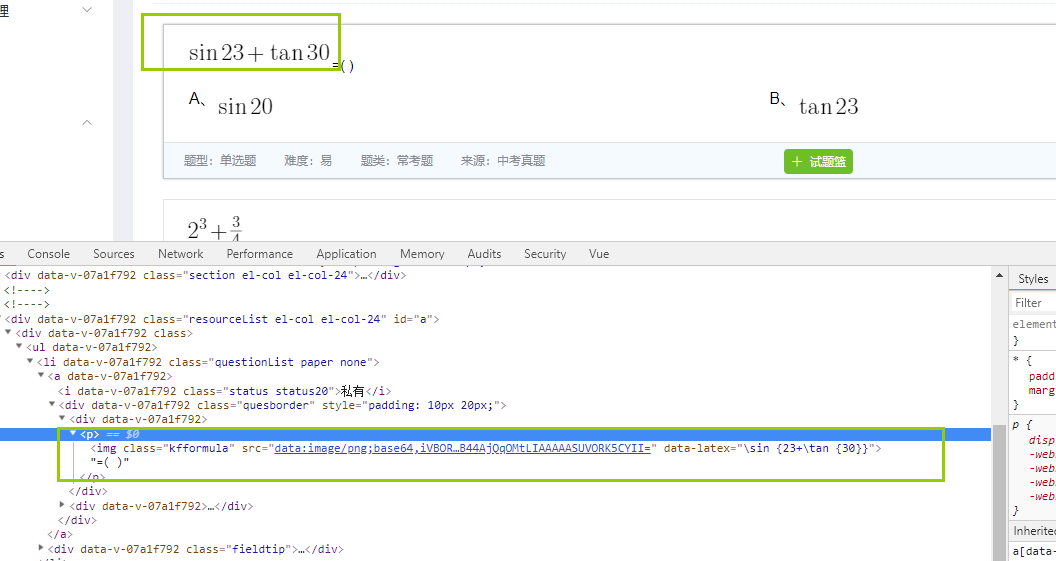这是个图片的公式，有img标签引入。

我们想要转成下面的效果：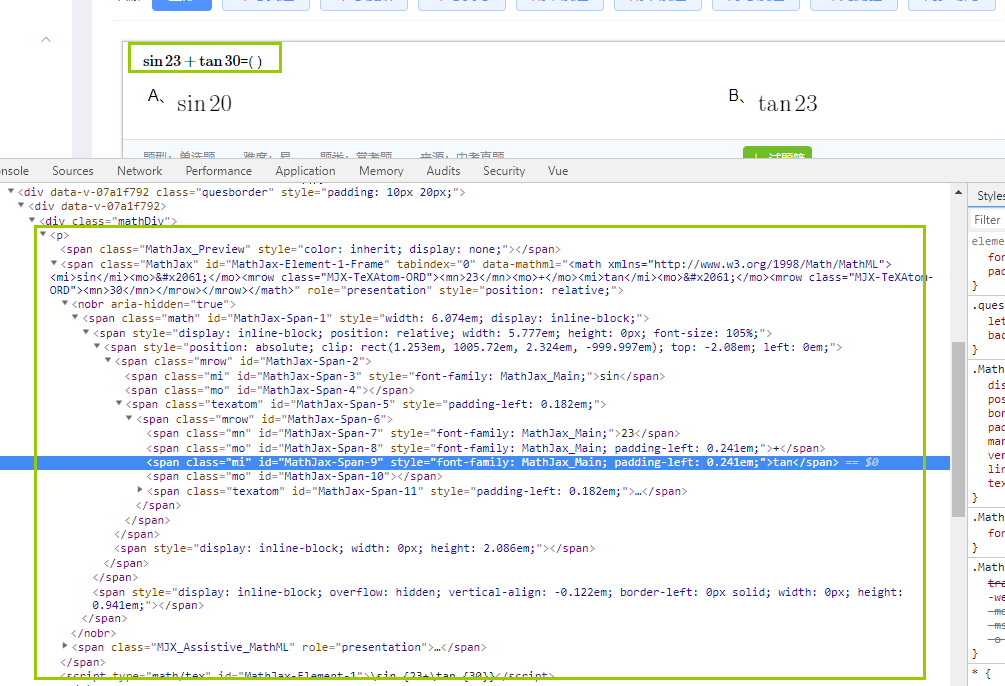在vue项目中引入MathJax.js

首先在index.html页面

<script type="text/javascript" src="https://cdn.mathjax.org/mathjax/latest/MathJax.js?config=TeX-AMS_HTML-full"></script>  <!--不使用图片显示数学公式插件-->这个是国际版的加载可能有点慢。推荐使用下面的

<script type="text/javascript" src="http://cdn.bootcss.com/mathjax/2.7.0/MathJax.js?config=TeX-AMS-MML_HTMLorMML"></script>  <!--不使用图片显示数学公式插件-->

因为有很多页面需要用到这个插件，所以我封装到了一个公共的js文件里面，想用的时候直接调用。

下面是自定义的util.js

export default {

//公式转换的配置文件
MathConfig(){

MathJax.Hub.Config({
showProcessingMessages: false,
messageStyle: "none",
extensions: ["tex2jax.js"],
jax: ["input/TeX", "output/HTML-CSS"],
tex2jax: {
inlineMath:  [ ["$", "$"] ],
displayMath: [ ["$$","$$"] ],
skipTags: ['script', 'noscript', 'style', 'textarea', 'pre','code','a'],
ignoreClass:"comment-content"
},
"HTML-CSS": {
availableFonts: ["STIX","TeX"],
}
});
},

//把图片公式替换成字符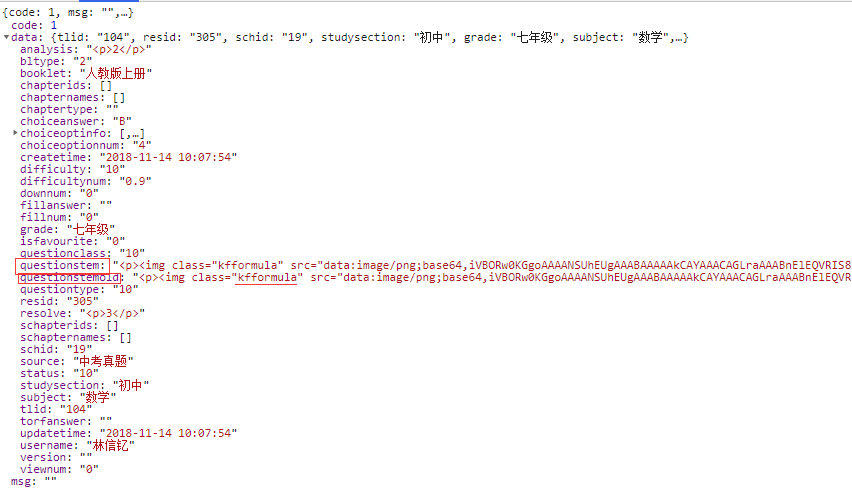resourceLists的数据内容
MathComputed(resourceLists){//resourceLists就是我想替换的里面可能有图片公式的内容。他如果是个数组直接用data接收，如果不是数组，push进data，让他成为数组。因为下面循环的所以这里必须放在数组里面。
var data=[];
if(resourceLists.length>0){data=resourceLists;}
else{data.push(resourceLists);}

for(var k=0;k<data.length;k++){//循环里面的题干内容

var html ='<div><div class="mathDiv">'+data[k].questionstem+'</div></div>';

var htmlObj = $(html); //将字符串转为jquery的dom对象 var filterObj = htmlObj.find(".kfformula"); //对转换后的对象进行查询 if(filterObj.length>0){//如果有公式。替换图片 for(var i=0;i<filterObj.length;i++){ var latex = '$' + $(filterObj[i]).attr('data-latex') + '$';
$(filterObj[i]).replaceWith(latex); } data[k].questionstem=htmlObj.html(); }else{//没有公式直接取值 data[k].questionstem=data[k].questionstem; } } } return data; }, }； 再具体的页面调用那写函数 import util from '../static/util.js' mounted() { util.MathConfig();//调用配置文件 }， //转换页面的图片数学公式 util.MathComputed(this.resourceLists); //这里是this.resourceLists是接口获取的要转化的内容 this.$nextTick(()=>{    //必须写这个表示dom元素都加载完了。
var mathdivs = $('#app').find(".mathDiv"); //对转换后的对象进行查询 for(var i=0;i<mathdivs.length;i++){ //必须循环然后才有作用，如果直接写#app不好用。所以这里就循环了 window.MathJax.Hub.Queue(["Typeset", MathJax.Hub,$(mathdivs[i])]);
}

})

展开全文• 本文主要介绍MathJax公式的基本语法。插入公式如果是在文本中插入公式，则用$...$。如果公式自成段落，则使用$$...$$。字体使用\mathbb或\Bbb来显示黑板粗体字，$\mathbb{NQRZ}$使用\mathbf来显示粗体字，$\mathbf {... 本文主要介绍MathJax公式的基本语法。 插入公式 如果是在文本中插入公式，则用$...$。 如果公式自成段落，则使用$$...$$。 字体使用\mathbb或\Bbb来显示黑板粗体字，$\mathbb{NQRZ}$使用\mathbf来显示粗体字，$\mathbf {ABCDabcd}$使用\mathtt来显示打印式字体，$\mathtt{ABCDabcd}$使用\mathrm来显示罗马字体，$\mathrm{ABCDabcd}$使用\mathcal来显示手写字体，$\mathcal{ABCDabcd}$使用\mathscr来显示剧本字体，$\mathscr{ABCDabcd}$使用\mathfrak来显示Fraktur字母(一种旧的德国字体)，$\mathfrak{ABCDabcd}$多行公式 如果要写出多行公式，就使用 \begin{equation}\begin{split} end{split}\end{equation} \\ 符号表示换行，再使用&符号表示要对齐的位置，例子如下 \begin{equation}\begin{split} H(Y|X)&=\sum_{x\in X} p(x)H(Y|X)\\ &=-\sum_{x\in X} p(x)\sum_{y\in Y}p(y|x)\log p(y|x)\\ &=-\sum_{x\in X} \sum_{y\in Y}p(y,x)\log p(y|x) \end{split}\end{equation} 当然，再加上$$...$$之后，显示如下： $$\begin{equation}\begin{split} H(Y|X)&=\sum_{x\in X} p(x)H(Y|X)\\ &=-\sum_{x\in X} p(x)\sum_{y\in Y}p(y|x)\log p(y|x)\\ &=-\sum_{x\in X} \sum_{y\in Y}p(y,x)\log p(y|x) \end{split}\end{equation}$$ 分组 通过大括号{}将操作数与符号分割开，消除二义性。 例如，若使用x^10，其效果为$x^10$，这里就要用到大括号，x^{10}，最终效果为$x^{10}$。 空间 MathJax通常用自己的一套复杂策略来决定公式的空间距离。直接在两个元素之间加入空格是毫无用处的。 因此为了增加空间距离，使用\,可以增加稍许空间；使用\;可以增加更多的空间；\quad和\qquad分别对应更大的空间。 数学符号 上标与下标 上标或下标只需在后面加上^或_。另外需要注意的是，如果上下标不止一个字符，就需要用大括号括起来，表示是一个整体{...}。 分式 有两种方式做到这个效果。 使用\frac ab。如\frac {1+a}{4+b}，效果为$\frac{1+a}{4+b}$; 使用a \over b。如{1+a} \over {4+b}，效果为${1+a}\over {4+b}$。 一般推荐使用第二种。个人倾向于使用第一种。 根式 平方根： \sqrt{x^3}，效果为$\sqrt{x^3} $。 其余： \sqrt{\frac xy}，效果为$\sqrt{\frac xy}$。 关系比较符号< \lt > \gt ≤ \le ≥ \ge ≠ \neq $$\begin{array}{c|lcr} \text{符号} & \text{\表示} \\ \hline < & lt \\ > & gt \\ ≤ & le \\ ≥ & ge \\ ≠ & neq \\ \end{array}$$ argmax\underset{f}{\mathrm{argmin}} 效果如下： $$\underset{f}{\mathrm{argmin}}$$ 不显示斜体 对于常量部分，有时候我们不想让他显示成斜体，而是正常的竖直显示，可加上\text{...}，就可以正常显示。 矩阵 使用$$\begin{matrix}…\end{matrix}$$，每一行末用\\结束表示换行，用&分隔矩阵元素。 $$\begin{matrix} 1 & 0 & 0 \\ 0 & 1 & 0 \\ 0 & 0 & 1 \\ \end{matrix}$$ 效果： $$\begin{matrix} 1 & 0 & 0 \\ 0 & 1 & 0 \\ 0 & 0 & 1 \\ \end{matrix}$$ 如果要加括号，可以使用上面的括号符号。 除此之外，还可以直接将matrix替换为pmatrix(小括号)，或者bmatrix(中括号)，或者Bmatrix(大括号)，或者vmatrix(竖线)，或者Vmatrix(是双竖线) 如果你想省略一些项，可以使用\cdots⋯，\ddots⋱，\vdots⋮。 如： $$\begin{pmatrix} 1 & a_1 & a_1^2 & \cdots & a_1^n\\ 1 & a_2 & a_2^2 & \cdots & a_2^n \\ \vdots & \vdots & \ddots & \vdots \\ 1 & a_n & a_n^2 & \cdots & a_n^n \\ \end{pmatrix}$$ 效果： $$\begin{pmatrix} 1 & a_1 & a_1^2 & \cdots & a_1^n\\ 1 & a_2 & a_2^2 & \cdots & a_2^n \\ \vdots & \vdots & \ddots & \vdots \\ 1 & a_n & a_n^2 & \cdots & a_n^n \\ \end{pmatrix}$$ 代码块 不同语言的代码块，可以用不同的标识符。如python语言，则代码块表示为 1//下一行写具体代码 就是用三个反引号(英文状态下、键盘左上角、ESC下面的那个键)包围的部分就是代码块，上面的三个点号之后，可以标识一下具体的语言，如c,c++,python,java,javascript,yml,html等等。 测试： JavaScript代码块效果如下： 1 2 3if (condition){ return true } Python代码块效果如下： 1 2 3def func(A): '''intresting document.''' return len(A) 展开全文• mathjax节点 该存储库包含一个提供API的库，该API可以从Node.js程序中调用 。 该API将单个数学表达式（采用MathJax的任何输入格式）转换为HTML（带有CSS），SVG或MathML代码。 利用 npm install mathjax-node 安装... • 然后在全局引入 也就是在main.js中 加入如图所示代码，具体代码如下 import Vue from 'vue' import App from './App.vue' // main.js import MathJax from './js/MathJax.js' // MathJax.js内容在1.3，位置随意 Vue.... 1.使用Webpakage新建一个简单的Vue项目 2.再新建项目的public目录下的index.html中，加入如下标签内容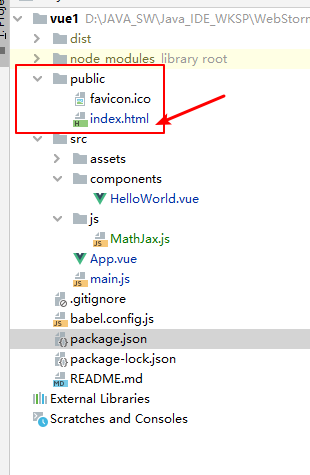整体MathJax的scriipt的引入 <!DOCTYPE html> <html lang=""> <head> <meta charset="utf-8"> <meta http-equiv="X-UA-Compatible" content="IE=edge"> <meta name="viewport" content="width=device-width,initial-scale=1.0"> <link rel="icon" href="<%= BASE_URL %>favicon.ico"> <title><%= htmlWebpackPlugin.options.title %></title> </head> <body> <noscript> <strong>We're sorry but <%= htmlWebpackPlugin.options.title %> doesn't work properly without JavaScript enabled. Please enable it to continue.</strong> </noscript> <div id="app"></div> <!-- "mathjax": "^3.1.4",--> </body> <script type="text/javascript" async src="https://cdnjs.cloudflare.com/ajax/libs/mathjax/2.7.5/MathJax.js?config=TeX-MML-AM_CHTML"></script> </html> 3.新建一个JS目录（随意），用来放初始化MathJax的脚本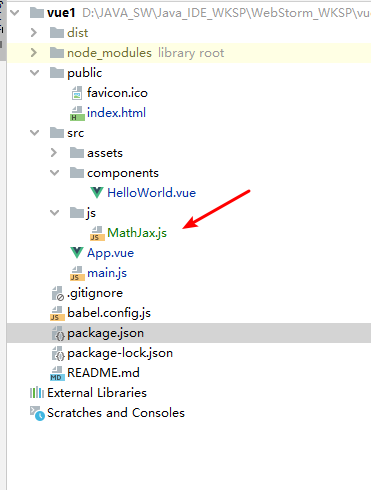里面的内容 如下 let isMathjaxConfig = false;//用于标识是否配置 const initMathjaxConfig = () => { if (!window.MathJax) { return; } window.MathJax.Hub.Config({ showProcessingMessages: false, //关闭js加载过程信息 messageStyle: "none", //不显示信息 jax: ["input/TeX", "output/HTML-CSS"], tex2jax: { inlineMath: [["$", "$"], ["\$$", "\$$"]], //行内公式选择符 displayMath: [["$$", "$$"], ["\$", "\$"]], //段内公式选择符 skipTags: ["script", "noscript", "style", "textarea", "pre", "code", "a"] //避开某些标签 }, "HTML-CSS": { availableFonts: ["STIX", "TeX"], //可选字体 showMathMenu: false //关闭右击菜单显示 } }); isMathjaxConfig = true; //配置完成，改为true }; const MathQueue = function (elementId) { if (!window.MathJax) { return; } window.MathJax.Hub.Queue(["Typeset", window.MathJax.Hub, document.getElementById(elementId)]); }; export default { isMathjaxConfig, initMathjaxConfig, MathQueue, } 4.然后在全局引入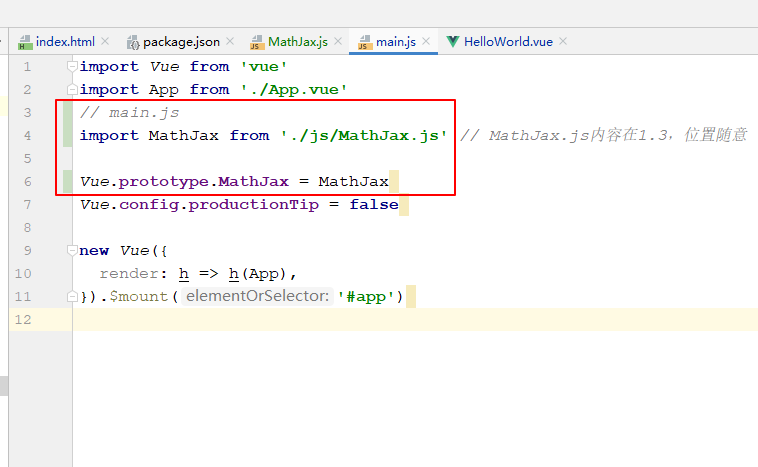也就是在main.js中 加入如图所示代码，具体代码如下 import Vue from 'vue' import App from './App.vue' // main.js import MathJax from './js/MathJax.js' // MathJax.js内容在1.3，位置随意 Vue.prototype.MathJax = MathJax Vue.config.productionTip = false new Vue({ render: h => h(App), }).$mount('#app')

5.最后就是使用，代码如下

<template xmlns:display="http://www.w3.org/1999/xhtml">
<div class="hello">
<div>{{text1}}</div>
</div>
</template>

<script>
import MathJax from '../js/MathJax'
export default {
name: 'HelloWorld',
props: {
msg: String
},
data(){
return{
text1 : '$$借款本金=\\sum _{nT}^{i=1}\\frac{第i期支付金额}{1+年化综合成本}$$'
}
},
methods: {
formatMath() {
let that = this;
setTimeout(function () {
• MathML转换成图片方法

千次阅读 2013-09-23 07:54:03脚本 MathML
• 在vue中渲染数学公式 - MathJax

千次阅读 2020-08-06 11:58:11
目前在使用 vue+ elementui做一个考试管理系统，在做学生考试页面时需要用到数学公式，但是后台解析出来后不是图片，而且如果是图片的话放在题目里很怪，所以需要使用到MathJax去解析后台传过来的公式 后台返回的...javascript vue js html 前端
• Markdown之列表&MathJax

千次阅读 2017-10-25 14:25:26
下面说的Markdown的表格形式已经被用烂了，但是一直不怎么用Markdown里面的MathJax，实在不行就小窗截图，随着这个审美的不断提升，现在看那个实在是越来越丑啊，下面贴下转载的帖子，好好学习下。1. 前言Markdown是......